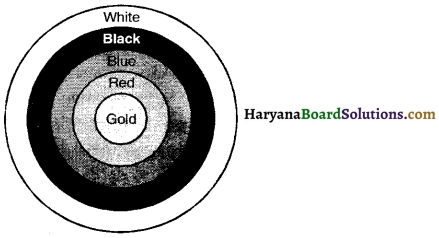# HBSE 10th Class Maths Solutions Chapter 12 Areas Related to Circles Ex 12.1

Haryana State Board HBSE 10th Class Maths Solutions Chapter 12 Areas Related to Circles Ex 12.1 Textbook Exercise Questions and Answers.

## Haryana Board 10th Class Maths Solutions Chapter 12 Areas Related to Circles Ex 12.1

Unless stated otherwise, use π = $$\frac{22}{7}$$.

Question 1.
The radii of two circles are 19 cm and 9 cm respectively. Find the radius of the circle which has circumference equal to the sum of the circumference of the two circles.
Solution.
Circumference of the circle of radius 19 cm = 2π × 19 = 38π cm
Circumference of the circle of radius 9 cm = 2π × 9 = 18π cm
Sum of the circumferences of two circles = 38π + 18π = 56π cm
Let R be the radius of the circle which has circumference equal to sum of the circumferences of the two circle.
2πR = 56π
⇒ R = $$\frac{56 \pi}{2 \pi}$$
⇒ R = 28 cm
Hence,radius of the circle = 28 cm.Question 2.
The radii of two circles are 8 cm and 6 cm respectively. Find the radius of the circle having area equal to the sum of the areas of the two circles.
Solution.
Area of circle of radius 8 cm = π × 82 = 64π cm2
Area of circle of radius 6 cm = π × 62 = 36π cm2
Sum of the areas of two circles = 64π + 36π = 100π cm2
Let R be the radius of the circle whose area is equal to the sum of the areas of the two circles.
⇒ πR2 = 100π
⇒ R2 = $$\frac{100 \pi}{\pi}$$ = 100
⇒ R = √100 = 10 cm
Hence, radius of the circle = 10 cm.Question 3.
Figure depicts an archery target marked with its five scoring regions from the centre outwards as Gold, Red, Blue, Black and White. The diameter of the region representing Gold score is 21 cm and each of the other bands is 10.5 cm wide. Find the area of the each of the five scoring regions.Solution.
We have,
Radius of region representing Gold score (r1) = $$\frac{21}{2}$$ = 10.5 cm
Area of region representing Gold score = π × (10.5)2
= $$\frac{22}{7}$$ × 10.5 × 10.5 = 346.5 cm2

Radius of region representing Red and Gold score areas (r2) = 10.5 + 10.5 = 21 cm
Area of region representing Red score = πr22 – πr12
= $$\frac{22}{7}$$ × (21)2 – 346.5
= 1386 – 346.5 = 1039.5 cm2

Radius of the region representing Blue, Red and Gold scoring areas (r3) = (21 + 10.5) cm = 31.5 cm
Area of region representing Blue scoring area = πr32 – (Area of Gold + Area of Red)
= $$\frac{22}{7}$$ × (31.5)2 – (346.5 + 1039.5)
= 3118.5 – 1386 = 1732.5 cm2

Radius of the region representing Black, Blue, Red and Gold scoring areas (r4) = (31.5 + 10.5) cm = 42 cm
Area of region representing Black scoring area = πr42 – (Area of Gold + Area of Red + Area of Blue)
= $$\frac{22}{7}$$ × (42)2 – (346.5 + 1039.5 + 1732.5)
= 5544 – 3118.5 = 2425.5 cm2

Radius of the region representing White, Black, Blue, Red and Gold, scoring areas (r5) = (42 + 10.5) cm = 52.5 cm.
Area of region representing White scoring area = πr52 – [Area of Gold + Area of Red + Area of Blue + Area of Black]
= $$\frac{22}{7}$$ × (52.5)2 – (346.5 + 1039.5 + 1732.5 + 2425.5)
= 8662.5 – 5544 = 3118.5 cm2

Hence, areas of Gold, Red, Blue, Black and White scoring regions are 346.5 cm2, 1039.5 cm2, 1732.5 cm2, 2425.5 cm2, and 3118.5 cm2 respectively.Question 4.
The wheels of a car are of diameter 80 cm each. How many complete revolutions does each wheel make in 10 minutes when the car is travelling at a speed of 66 km/hour ?
Solution.
We have,
Speed of car = 66 km/hour
Distance travelled by car in 1 minute = $$\frac{66}{100}$$ × 1000 × 100 = 110000 cm
Distance travelled by car in 10 minutes = 110000 × 10 = 1100000 cm
Radius of car’s wheel = $$\frac{80}{2}$$ = 40 cm
Circumference of wheel = 2πr
= 2 × $$\frac{22}{7}$$ × 40 = $$\frac{1760}{7}$$ cm
Distance covered by the wheel of a car in 1 revolution = $$\frac{1760}{7}$$ cm
Number of revolutions made by the wheel of a car in 10 minutes = $$\frac{\text { Distance travelled by car in } 10 \text { minutes }}{\text { Distance travelled by car in1 revolution }}$$
= $$\frac{1100000}{\frac{1760}{7}}$$
= $$\frac{1100000 \times 7}{1760}$$
= 4375
Hence, number of revolutions made by wheel of a car = 4375.Question 5.
Tick the correct answer in the following and justify your choice: If the perimeter and the area of a circle are numerically equal, then the radius of the circle is
(A) 2 units
(B) π units
(C) 4 units
(D) 7 units
Solution.
Correct option is (A)
Justification. Let the radius of circle is r units.
Acoording to questions
πr2 = 2πr
$$\frac{\pi r^2}{\pi r}$$ = 2
r = 2.